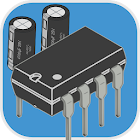Electronics Toolbox

All Android applications categories

All Android games categories# Electronics Toolbox

by: 723 8.8

8.8 Users
rating

## Screenshots

Description

This app is a collection of basic electronic calculators. It is suitable for hobbyist, electronic engineers or professionals.

This is the free version, which contains ads; you can also buy from the Market the PRO version of the app to support the developer, unlock more features and get rid of the ads.

Features
• Calculate resistance of 2 resistors in series / parallel
• To find out combinations of 2 resistors in series / in parallel making a desired resistance
• Calculate resistor values of voltage divider and find out combinations of resistance making a desired ratio
• Calculate resistor values of inverting amplifier gain and find out combinations of resistance making a desired ratio
• Ohm's Law calculation
• Star-delta conversion
• RC charging circuit : Calculate time constant by R and C
• RC Filter : Calculate cutoff frequency by resistor and capacitor value and find out combinations of resistance and capacitance making a desired cutoff frequency
• RL Filter : Calculate cutoff frequency by resistor and inductor value and find out combinations of resistance and inductance making a desired cutoff frequency
• LC Filter : Calculate resonant frequency by capacitor and inductor values and find out combinations of capacitance and inductance making a desired resonant frequency
• Calculate monostable circuit
• Calculate frequency of astable circuit and find out combinations of capacitance and resistance making a desired astable frequency

Features in PRO version only:
2. No limitation
Pro version :